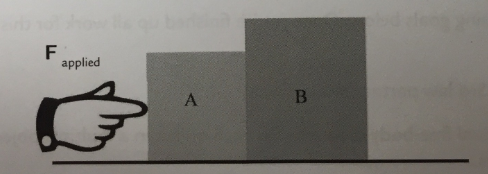# Problem: 3. Two blocks (A and B)  are in contact on a horizontal frictionless surface. A 40 N constant horizontal force is applied to A. The mass of A is 3.0 kg and the mass of B is 12 kg. What is the magnitude of the force of A on B?

###### FREE Expert Solution

Newton's second law,

$\overline{){\mathbf{\Sigma F}}{\mathbf{=}}{\mathbf{m}}{\mathbf{a}}}$

Block A applies/transmits a force FB to block B. Sum of forces on block B:

ΣFB = FB = mBaB

Block A experiences the applied force and reaction force from Block B. The reaction force from block B is equal to FB directed opposite to the applied force.

ΣFA = Fappied - FB = mAaA

Fapplied = FB + mAaA =  mBaB + mAaA

aA = aB = a

85% (102 ratings)###### Problem Details

3. Two blocks (A and B)  are in contact on a horizontal frictionless surface. A 40 N constant horizontal force is applied to A. The mass of A is 3.0 kg and the mass of B is 12 kg. What is the magnitude of the force of A on B?